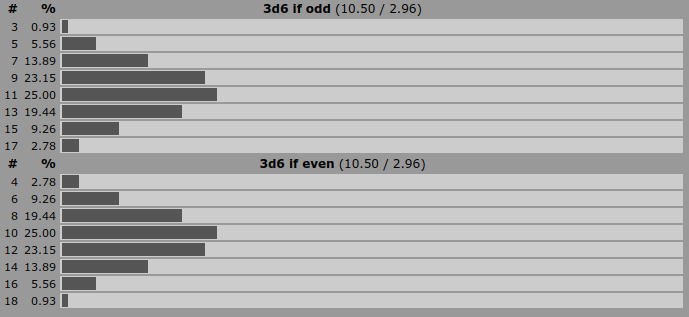# [RPG] How to calculate conditional probabilities in AnyDice

anydicestatistics

While writing the addendum to this answer, which considers the relative value of skill vs. characteristic in the "3d20 system" of Neuroshima, I found myself wanting an answer to a deceptively simple question: how many skill points are needed to succeed if the lowest roll is a natural success, vs. if it's not? In other words, I basically wanted to plot the distributions of:

• the middle roll of 3d20, given that the lowest roll is less than some given threshold x; and
• the sum of the lowest and middle rolls, given that the lowest roll is at least x.

In statistics, this would be just a standard conditional probability distribution, e.g. $$p_x(y) = P(Y = y \mid X < x),$$ $$q_x(z) = P(X + Y = z \mid X \ge x),$$ where $$\X\$$ and $$\Y\$$ are (interdependent) random variables representing the lowest and the middle roll of 3d20 respectively. You could compute this easily just by taking the joint distribution of $$\(X,Y)\$$, dropping those cases where the condition (e.g. $$\X < x\$$) fails, rescaling the remaining probabilities so that they sum to 1 and then optionally summing over the conditioning variable $$\X\$$ to obtain the marginal distribution of $$\Y\$$ (or $$\X + Y\$$).

Unfortunately, there seems to be no simple built-in way to do this in AnyDice. In fact, there doesn't even seem to be any way to answer simpler conditional probability questions like, say "what is the average sum of 3d6 if the sum rolled is even, vs. if it's odd?"

So, hence this question: Is there any way to calculate a conditional probability distribution in AnyDice, and if so, how?

Disclaimer: I realize that this question may be borderline off-topic for this site, as it's more of a programming / math question. That said, it did arise in an RPG-related context — specifically, while writing an answer here on RPG.SE — and I suspect the answer(s) may be useful to others using AnyDice to answer similar questions about other systems as well. I'll let the community decide if this Q&A should stay here or not.

Also, I did eventually manage to come up with a (slightly hacky but workable) solution to my problem on my own, so I've posted a self-answer below. That said, other answers are more than welcome too. If there's a better way to achieve this, I would very much like to know it.

## Use the "empty die" result to disregard cases that don't meet criteria

If we want to completely disregard a certain subset of results, we can do this by using a function which returns the "empty die", `d{}`, for cases which do not meet our desired conditions.

The empty die `d{}` appears to be a special die which has no possible results and no associated probability. Consequently, if we define a function which returns this empty die for certain input cases, it is effectively removing those cases from the set of possible results, and the result distribution that comes back from the function is as if the unwanted cases were never invoked.

Here's a simple function which simply limits the received input to a set of allowed values and discards cases which don't satisfy that condition:

``````function: if X:n in RESTRICT:s {
if X = RESTRICT { result: X }
result: d{}
}
``````

Given an input `X`, if `X` can be found in the sequence of allowed values `RESTRICT`, all is well and we return `X`; otherwise, we return `d{}`, assigning zero probability to that particular outcome. We can use this function to restrict a 3d6 roll to only odd or even values:

``````output [if 3d6 in {3,5,7,9,11,13,15,17}] named "3d6 if odd"
output [if 3d6 in {4,6,8,10,12,14,16,18}] named "3d6 if even"
``````

And we get a result that looks like this:This obviously extends to more interesting cases, such as the Neuroshima rules given in the question. Here's a program which shows examples of those distributions:

``````function: INDEX:s at DICE:s if lowest less than MIN:n {
if (#DICE@DICE >= MIN) { result: d{} }
result: INDEX@DICE
}

function: INDEX:s at DICE:s if lowest at least MIN:n {
if (#DICE@DICE < MIN) { result: d{} }
result: INDEX@DICE
}

MIN: 10

output [2 at 3d20 if lowest less than MIN] named "Middle die of 3d20 if lowest die less than [MIN]"
output [{2,3} at 3d20 if lowest at least MIN] named "Middle and lowest die of 3d20 if lowest die at least [MIN]"
``````

These functions first discard cases which don't meet the specified condition and then give us the values we care about from the remaining dice sequences.

You could of course also approach that problem the other way round and define functions which map undesired results to a bogus value (like -1) and then pipe that through a filtering function at the end which strips out any results with the bogus value, though doing the filtering as early as possible is I think more efficient in Anydice and will probably let you get away with running more complex programs/larger dice pools.

## Background

I hit upon this empty die trick while working on this answer to another question. Essentially, I wrote a simple function that would recursively reroll 4d6-droplow until it got an 8 or better, but I realised on inspection that the result distribution it returned didn't change no matter what I set the maximum function depth to.

In Anydice, as the documentation says, exceeding the maximum function depth simply causes the function to return the empty die, and I figured out from there that meant the empty die was essentially a zero-probability result which does not affect the final result distribution, and that we can return it on purpose (rather than accidentally by exceeding function depth) if we want to disregard some category of inputs!